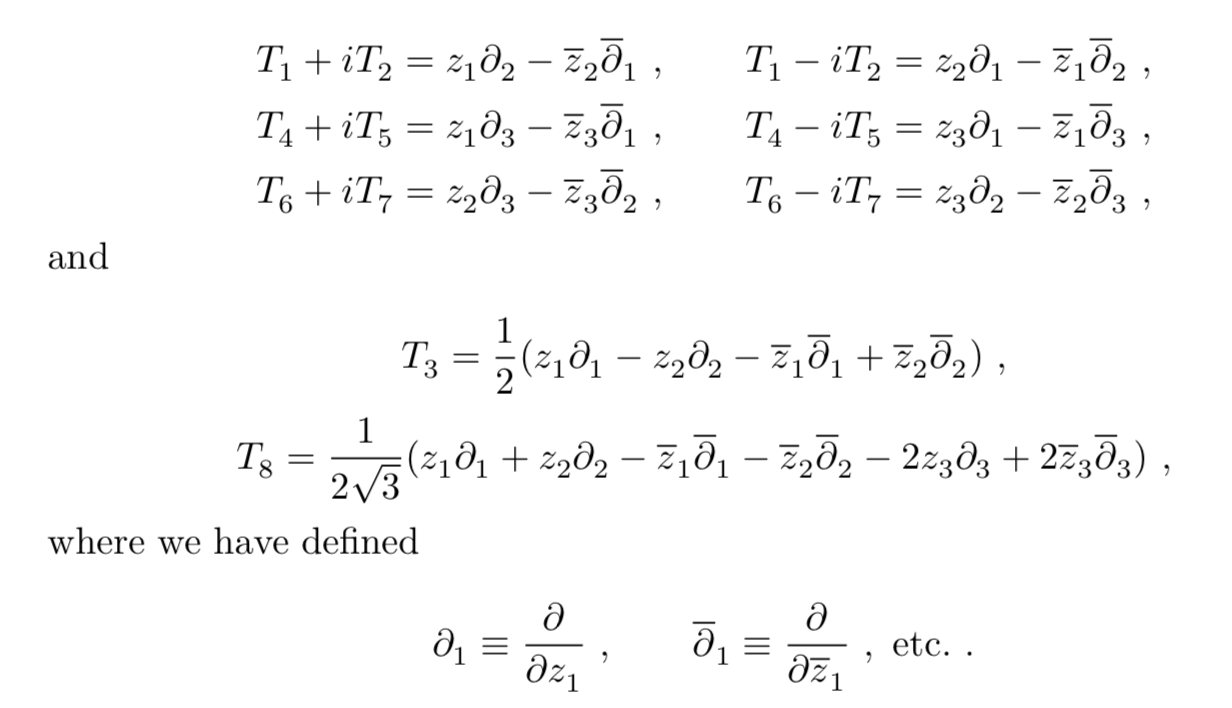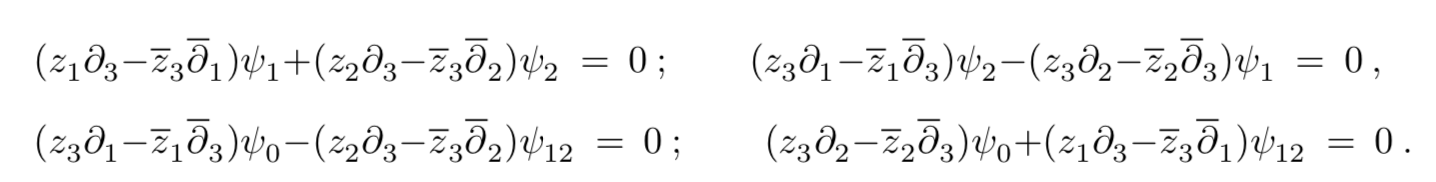Definition of a Dirac operator

So it seems that a Dirac operator acting on spinors on $$\psi=\psi(\mathfrak{su}(2),\mathbb{C}^2)$$ can be written in this case simply as:

$$D=\sum_{i,j} E_{ij}\otimes e_{ji}$$, where $$E_{ij}$$ are elements of $$U(\mathfrak{g})$$ and $$e_{ji}$$ are elements of a Clifford algebra.

In the easiest form, $$D=\sigma_x\partial_x+\sigma_y\partial_y+\sigma_z\partial_z$$, where sigmas are the Pauli matrices for $$su(2)$$ on Euclidean space.

There's a cubic Dirac operator by Kostant, it reads something like $$D=\sum_{i,j} E_{ij}\otimes e_{ji}+\sum_{ijkl}^{(2)}\otimes (e_{ij}\wedge e_{kl})$$ but I am a bit unsure of what's $$E_{ijkl}$$ in this case, and how it is constructed. Could anyone write it "in the easiest form" as I did for $$su(2)$$?

For higher order, I'm guessing it is written in the exterior algebra of $$\mathbb{C}^n$$. Do you have any clue of matrices are these and how they are constructed?

Thank you!

• Could you provide a reference, or better a link? Mar 12 '19 at 4:49
• See page 23 of web.math.ucsb.edu/~dai/book.pdf. Mar 12 '19 at 20:21

An explicit representation of Kostant's equation over $$SU(3)/SU(2)\times U(1)$$ is given in Supersymmetry and Euler Multiplets. It is given in terms of Dirac matrices $$\{\gamma^a,\gamma^b\}=2\delta^{ab},\;\;a,b=4,5,6,7$$ and $$SU(3)$$ generators $$T_n$$, $$n=1,2,\ldots 8$$ by $$\sum_{a=4,5,6,7}\gamma^a T_a\Psi=0$$ where the generators have the following representation as differential operators: